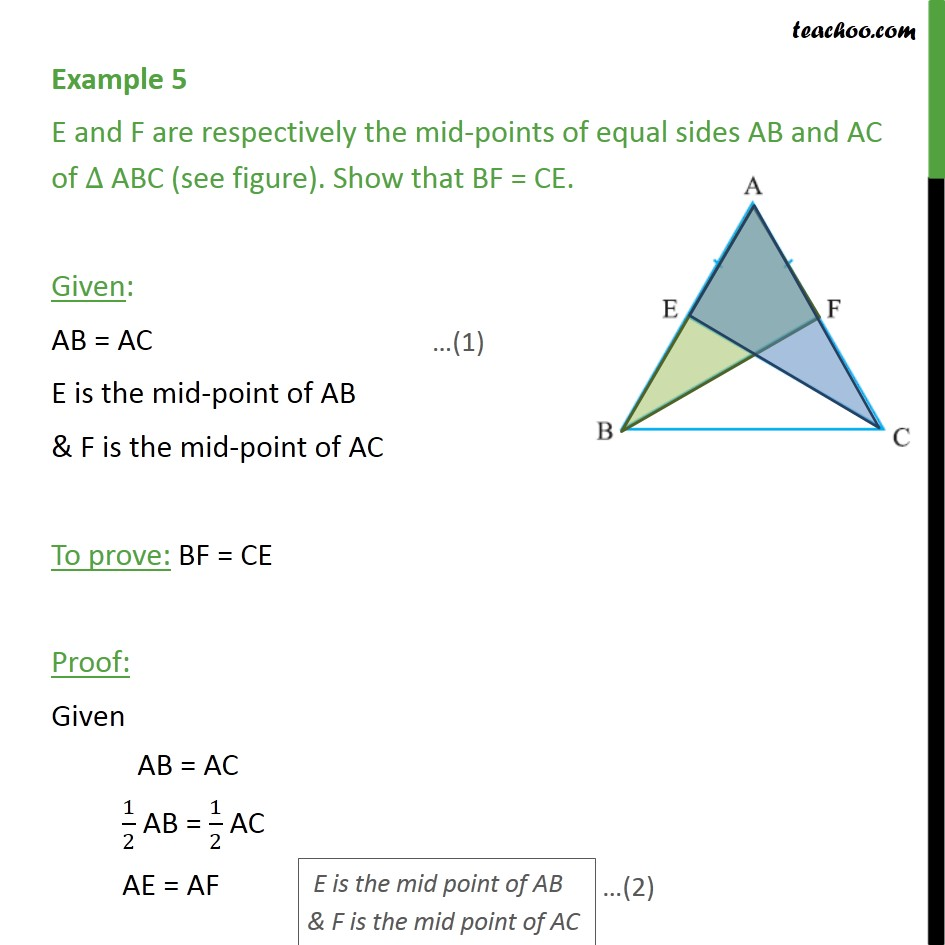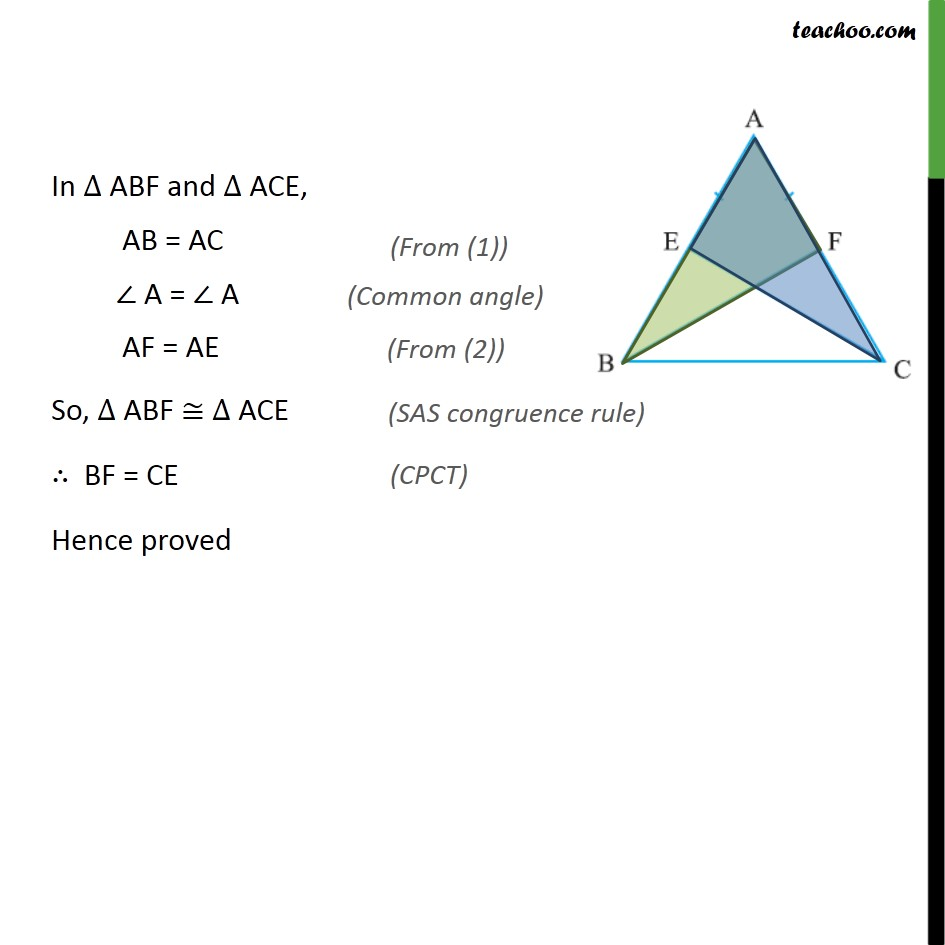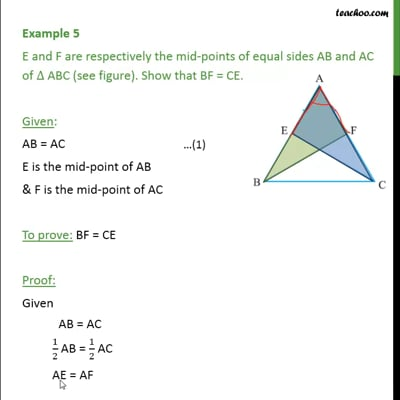Examples

Chapter 7 Class 9 Triangles
Serial order wiseThis video is only available for Teachoo black users

Learn in your speed, with individual attention - Teachoo Maths 1-on-1 Class

### Transcript

Example 5 E and F are respectively the mid-points of equal sides AB and AC of ABC (see figure). Show that BF = CE. Given: AB = AC E is the mid-point of AB & F is the mid-point of AC To prove: BF = CE Proof: Given AB = AC 1/2 AB = 1/2 AC AE = AF In ABF and ACE, AB = AC A = A AF = AE So, ABF ACE BF = CE Hence proved